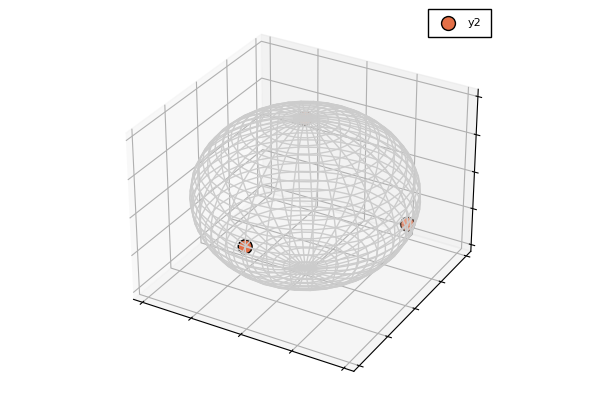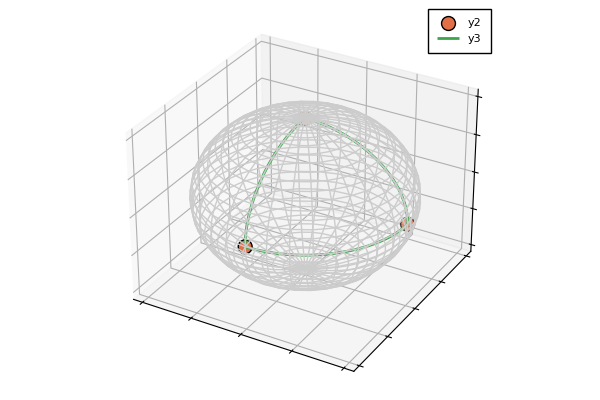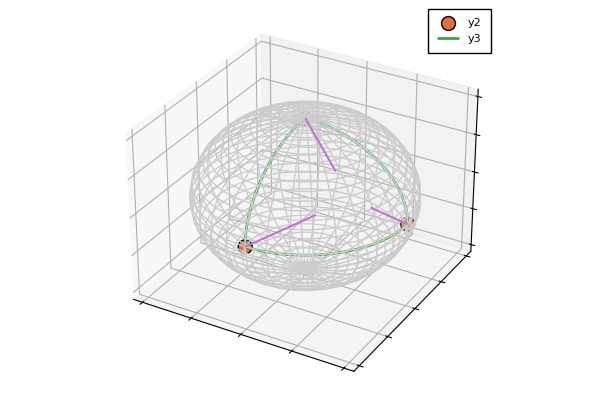# Sphere and unit norm arrays

Manifolds.AbstractSphereType
AbstractSphere{𝔽} <: AbstractDecoratorManifold{𝔽}

An abstract type to represent a unit sphere that is represented isometrically in the embedding.

source

The classical sphere, i.e. unit norm (real- or complex-valued) vectors can be generated as usual: to create the 2-dimensional sphere (in $ℝ^3$), use Sphere(2) and Sphere(2,ℂ), respectively.

Manifolds.SphereType
Sphere{n,𝔽} <: AbstractSphere{𝔽}

The (unit) sphere manifold $𝕊^{n}$ is the set of all unit norm vectors in $𝔽^{n+1}$. The sphere is represented in the embedding, i.e.

$$$𝕊^{n} := \bigl\{ p \in 𝔽^{n+1}\ \big|\ \lVert p \rVert = 1 \bigr\}$$$

where $𝔽\in\{ℝ,ℂ,ℍ\}$. Note that compared to the ArraySphere, here the argument n of the manifold is the dimension of the manifold, i.e. $𝕊^{n} ⊂ 𝔽^{n+1}$, $n\in ℕ$.

The tangent space at point $p$ is given by

$$$T_p𝕊^{n} := \bigl\{ X ∈ 𝔽^{n+1}\ |\ \Re(⟨p,X⟩) = 0 \bigr \},$$$

where $𝔽\in\{ℝ,ℂ,ℍ\}$ and $⟨\cdot,\cdot⟩$ denotes the inner product in the embedding $𝔽^{n+1}$.

For $𝔽=ℂ$, the manifold is the complex sphere, written $ℂ𝕊^n$, embedded in $ℂ^{n+1}$. $ℂ𝕊^n$ is the complexification of the real sphere $𝕊^{2n+1}$. Likewise, the quaternionic sphere $ℍ𝕊^n$ is the quaternionification of the real sphere $𝕊^{4n+3}$. Consequently, $ℂ𝕊^0$ is equivalent to $𝕊^1$ and Circle, while $ℂ𝕊^1$ and $ℍ𝕊^0$ are equivalent to $𝕊^3$, though with different default representations.

This manifold is modeled as a special case of the more general case, i.e. as an embedded manifold to the Euclidean, and several functions like the inner product and the zero_vector are inherited from the embedding.

Constructor

Sphere(n[, field=ℝ])

Generate the (real-valued) sphere $𝕊^{n} ⊂ ℝ^{n+1}$, where field can also be used to generate the complex- and quaternionic-valued sphere.

source

For the higher-dimensional arrays, for example unit (Frobenius) norm matrices, the manifold is generated using the size of the matrix. To create the unit sphere of $3×2$ real-valued matrices, write ArraySphere(3,2) and the complex case is done – as for the Euclidean case – with an keyword argument ArraySphere(3,2; field = ℂ). This case also covers the classical sphere as a special case, but you specify the size of the vectors/embedding instead: The 2-sphere can here be generated ArraySphere(3).

Manifolds.ArraySphereType
ArraySphere{T<:Tuple,𝔽} <: AbstractSphere{𝔽}

The (unit) sphere manifold $𝕊^{n₁,n₂,...,nᵢ}$ is the set of all unit (Frobenius) norm elements of $𝔽^{n₁,n₂,...,nᵢ}$, where 𝔽\in{ℝ,ℂ,ℍ}. The generalized sphere is represented in the embedding, and supports arbitrary sized arrays or in other words arbitrary tensors of unit norm. The set formally reads

$$$𝕊^{n_1, n_2, …, n_i} := \bigl\{ p \in 𝔽^{n_1, n_2, …, n_i}\ \big|\ \lVert p \rVert = 1 \bigr\}$$$

where $𝔽\in\{ℝ,ℂ,ℍ\}$. Setting $i=1$ and $𝔽=ℝ$ this simplifies to unit vectors in $ℝ^n$, see Sphere for this special case. Note that compared to this classical case, the argument for the generalized case here is given by the dimension of the embedding. This means that Sphere(2) and ArraySphere(3) are the same manifold.

The tangent space at point $p$ is given by

$$$T_p 𝕊^{n_1, n_2, …, n_i} := \bigl\{ X ∈ 𝔽^{n_1, n_2, …, n_i}\ |\ \Re(⟨p,X⟩) = 0 \bigr \},$$$

where $𝔽\in\{ℝ,ℂ,ℍ\}$ and $⟨\cdot,\cdot⟩$ denotes the (Frobenius) inner product in the embedding $𝔽^{n_1, n_2, …, n_i}$.

This manifold is modeled as an embedded manifold to the Euclidean, i.e. several functions like the inner product and the zero_vector are inherited from the embedding.

Constructor

ArraySphere(n₁,n₂,...,nᵢ; field=ℝ)

Generate sphere in $𝔽^{n_1, n_2, …, n_i}$, where $𝔽$ defaults to the real-valued case $ℝ$.

source

There is also one atlas available on the sphere.

Manifolds.StereographicAtlasType
StereographicAtlas()

The stereographic atlas of $S^n$ with two charts: one with the singular point (-1, 0, ..., 0) (called :north) and one with the singular point (1, 0, ..., 0) (called :south).

source

## Functions on unit spheres

Base.expMethod
exp(M::AbstractSphere, p, X)

Compute the exponential map from p in the tangent direction X on the AbstractSphere M by following the great arc eminating from p in direction X.

$$$\exp_p X = \cos(\lVert X \rVert_p)p + \sin(\lVert X \rVert_p)\frac{X}{\lVert X \rVert_p},$$$

where $\lVert X \rVert_p$ is the norm on the tangent space at p of the AbstractSphere M.

source
Base.logMethod
log(M::AbstractSphere, p, q)

Compute the logarithmic map on the AbstractSphere M, i.e. the tangent vector, whose geodesic starting from p reaches q after time 1. The formula reads for $x ≠ -y$

$$$\log_p q = d_{𝕊}(p,q) \frac{q-\Re(⟨p,q⟩) p}{\lVert q-\Re(⟨p,q⟩) p \rVert_2},$$$

and a deterministic choice from the set of tangent vectors is returned if $x=-y$, i.e. for opposite points.

source
Manifolds.local_metricMethod
local_metric(M::Sphere{n}, p, ::DefaultOrthonormalBasis)

return the local representation of the metric in a DefaultOrthonormalBasis, namely the diagonal matrix of size $n×n$ with ones on the diagonal, since the metric is obtained from the embedding by restriction to the tangent space $T_p\mathcal M$ at $p$.

source
ManifoldsBase.distanceMethod
distance(M::AbstractSphere, p, q)

Compute the geodesic distance betweeen p and q on the AbstractSphere M. The formula is given by the (shorter) great arc length on the (or a) great circle both p and q lie on.

$$$d_{𝕊}(p,q) = \arccos(\Re(⟨p,q⟩)).$$$
source
ManifoldsBase.get_coordinatesMethod
get_coordinates(M::AbstractSphere{ℝ}, p, X, B::DefaultOrthonormalBasis)

Represent the tangent vector X at point p from the AbstractSphere M in an orthonormal basis by rotating the hyperplane containing X to a hyperplane whose normal is the $x$-axis.

Given $q = p λ + x$, where $λ = \operatorname{sgn}(⟨x, p⟩)$, and $⟨⋅, ⋅⟩_{\mathrm{F}}$ denotes the Frobenius inner product, the formula for $Y$ is

$$$\begin{pmatrix}0 \\ Y\end{pmatrix} = X - q\frac{2 ⟨q, X⟩_{\mathrm{F}}}{⟨q, q⟩_{\mathrm{F}}}.$$$
source
ManifoldsBase.get_vectorMethod
get_vector(M::AbstractSphere{ℝ}, p, X, B::DefaultOrthonormalBasis)

Convert a one-dimensional vector of coefficients X in the basis B of the tangent space at p on the AbstractSphere M to a tangent vector Y at p by rotating the hyperplane containing X, whose normal is the $x$-axis, to the hyperplane whose normal is p.

Given $q = p λ + x$, where $λ = \operatorname{sgn}(⟨x, p⟩)$, and $⟨⋅, ⋅⟩_{\mathrm{F}}$ denotes the Frobenius inner product, the formula for $Y$ is

$$$Y = X - q\frac{2 \left\langle q, \begin{pmatrix}0 \\ X\end{pmatrix}\right\rangle_{\mathrm{F}}}{⟨q, q⟩_{\mathrm{F}}}.$$$
source
ManifoldsBase.inverse_retractMethod
inverse_retract(M::AbstractSphere, p, q, ::ProjectionInverseRetraction)

Compute the inverse of the projection based retraction on the AbstractSphere M, i.e. rearranging $p+X = q\lVert p+X\rVert_2$ yields since $\Re(⟨p,X⟩) = 0$ and when $d_{𝕊^2}(p,q) ≤ \frac{π}{2}$ that

$$$\operatorname{retr}_p^{-1}(q) = \frac{q}{\Re(⟨p, q⟩)} - p.$$$
source
ManifoldsBase.parallel_transport_toMethod
parallel_transport_to(M::AbstractSphere, p, X, q)

Compute the parallel transport on the Sphere of the tangent vector X at p to q, provided, the geodesic between p and q is unique. The formula reads

$$$P_{p←q}(X) = X - \frac{\Re(⟨\log_p q,X⟩_p)}{d^2_𝕊(p,q)} \bigl(\log_p q + \log_q p \bigr).$$$
source
ManifoldsBase.projectMethod
project(M::AbstractSphere, p, X)

Project the point X onto the tangent space at p on the Sphere M.

$$$\operatorname{proj}_{p}(X) = X - \Re(⟨p, X⟩)p$$$
source
ManifoldsBase.projectMethod
project(M::AbstractSphere, p)

Project the point p from the embedding onto the Sphere M.

$$$\operatorname{proj}(p) = \frac{p}{\lVert p \rVert},$$$

where $\lVert\cdot\rVert$ denotes the usual 2-norm for vectors if $m=1$ and the Frobenius norm for the case $m>1$.

source
ManifoldsBase.retractMethod
retract(M::AbstractSphere, p, X, ::ProjectionRetraction)

Compute the retraction that is based on projection, i.e.

$$$\operatorname{retr}_p(X) = \frac{p+X}{\lVert p+X \rVert_2}$$$
source

## Visualization on Sphere{2,ℝ}

You can visualize both points and tangent vectors on the sphere.

Note

There seems to be no unified way to draw spheres in the backends of Plots.jl. This recipe currently uses the seriestype wireframe and surface, which does not yet work with the default backend GR.

In general you can plot the surface of the hyperboloid either as wireframe (wireframe=true) additionally specifying wires (or wires_x and wires_y) to change the density of the wires and a wireframe_color for their color. The same holds for the plot as a surface (which is false by default) and its surface_resolution (or surface_resolution_lat or surface_resolution_lon) and a surface_color.

using Manifolds, Plots
pyplot()
M = Sphere(2)
pts = [ [1.0, 0.0, 0.0], [0.0, -1.0, 0.0], [0.0, 0.0, 1.0], [1.0, 0.0, 0.0] ]
scene = plot(M, pts; wireframe_color=colorant"#CCCCCC", markersize=10)which scatters our points. We can also draw connecting geodesics, which here is a geodesic triangle. Here we discretize each geodesic with 100 points along the geodesic. The default value is geodesic_interpolation=-1 which switches to scatter plot of the data.

plot!(scene, M, pts; wireframe=false, geodesic_interpolation=100, linewidth=2)And we can also add tangent vectors, for example tangents pointing towards the geometric center of given points.

pts2 =  [ [1.0, 0.0, 0.0], [0.0, -1.0, 0.0], [0.0, 0.0, 1.0] ]
p3 = 1/sqrt(3) .* [1.0, -1.0, 1.0]
vecs = log.(Ref(M), pts2, Ref(p3))
plot!(scene, M, pts2, vecs; wireframe = false, linewidth=1.5)